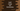# How to merge two Dictionaries in swiftBy merging two swift dictionaries, we can add the contents. Merging is straight forward. The only problem is conflicting keys. If we have same keys in both dictionaries, we need to specify which one to pick.

Swift provides two methods merge() and merging() for easy dictionary merge. I will show you how to use these methods with examples in this post. The only difference between these methods is that the former merges in-place and **the later one returns a new dictionary.

### Resolving key conflicts :

merge() and merging() methods takes one closure as the second parameter. This closure will decide which key to select in case of any conflicts.

### Example of merge() :

merge() takes two parameters. The first one is the dictionary and the second one is a closure that takes the current and new value for any duplicate key and returns the desired value.

As I told you before, it merges in place. For example :

``````var dict_one = ["first_no" : 1, "second_no" : 2]
var dict_two = ["second_no" : 3, "third_no" : 4]

dict_one.merge(dict_two){(first, _) in first}

print(dict_one)``````

This example will merge dict_one with dict_two and place that result in dict_one. It will print the below output :

**

``["third_no": 4, "first_no": 1, "second_no": 2]``

Here, we are taking the value of dict_one if the keys of both dict_one and dict_two are equal.

We can also change this example to take the value from the second dictionary dict_two :

``````var dict_one = ["first_no" : 1, "second_no" : 2]
var dict_two = ["second_no" : 3, "third_no" : 4]

dict_one.merge(dict_two){(_, second) in second}

print(dict_one)``````

It will print :

``["first_no": 1, "second_no": 3, "third_no": 4]``

### Example of merging :

merging is similar to merge. It takes the exact two parameters. The only difference is that it returns one new dictionary combining both dictionaries. For example :

``````var dict_one = ["first_no" : 1, "second_no" : 2]
var dict_two = ["second_no" : 3, "third_no" : 4]

var dict_result = dict_one.merging(dict_two){(_, second) in second}

print(dict_result)``````

Merging returned the result and we are storing it in dict_result. It will print :

``["first_no": 1, "second_no": 3, "third_no": 4]``

merging is useful if you don’t want in-place merging.